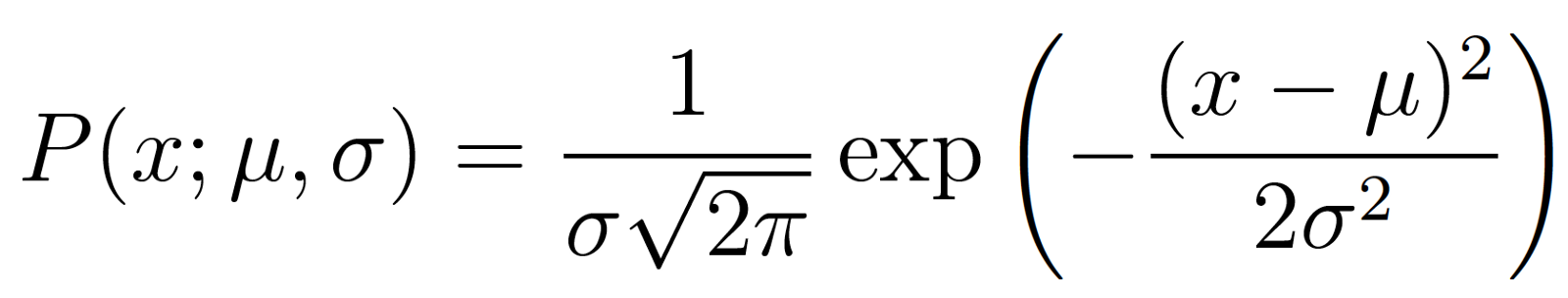# LOGISTIC REGRESSION

#### What is logistic regression?

Logistic regression a classification algorithm used for binary classification given a set of independent variables.
The output variables will be categorical like (yes/no), (0 or 1) etc. it predicts the probability of occurrence of an event by fitting data to a logit function.

logistic regression produces a logistic curve, which is limited to values between 0 and 1.
logistic regression uses maximum likelihood estimation (MLE) to obtain the model coefficients that relate predictors to the target. After this initial function is estimated, the process is repeated until LL (Log Likelihood) does not change.

Its called Regression but performs classification as based on the regression it classifies the dependent variable into either of the classes.

#### Classification with Logistic Regression

A classifier is a machine learning model that is used to discriminate different objects based on certain features.
Classification is the process of predicting a qualitative response. Methods used for classification often predict the probability of each of the categories of a qualitative variable as the basis for making the classification
There are many classification methods like k nearest neighbor, decision tree, Bayes classifier, neural network.

k nearest neighbor:
KNN can be used for both classification and regression predictive problems. It is more widely used in classification problems. The k in knn is the number of observations to be considered for classification with a specific data point so that we can categorize the data point in that category.

Decision tree:
Decision tree is used for classification and prediction. Decision tree is like a tree structure, where each internal node denotes a test on an attribute, each branch represents an outcome of the test, and each leaf node (terminal node) holds a class label. Decision trees classify instances by sorting them down the tree from the root to some leaf node, which provides the classification of the instance

Naïve Bayes classifier:
A Naive Bayes classifier is a probabilistic machine learning model that’s used for classification task. The most important fact of the classifier is based on the Bayes theorem.Using Bayes theorem, we can find the probability of A happening, given that B has occurred. Here, B is the evidence and A is the hypothesis. The assumption made here is that the predictors/features are independent. That is presence of one particular feature does not affect the other.

Neural network:
Neural network is a algorithm that mimics the action of the human brain ,like human brain it has number of neurons connecting each other to process the information The network consists of simple processing elements that are interconnected via weights
Neural networks help us cluster and classify. You can think of them as a clustering and classification layer on top of the data you store and manage. They help to group unlabeled data according to similarities among the example inputs, and they classify data when they have a labeled dataset to train on.

#### Maximum Likelihood Estimation

MLE can be defined as a method for estimating population parameters (such as the mean and variance for Normal, rate (lambda) for Poisson, etc.) from sample data such that the probability (likelihood) of obtaining the observed data is maximized.
MLE is the technique which helps us in determining the parameters of the distribution that best describe the given data.Maximum likelihood estimation is a method that determines values for the parameters of a model. The parameter values are found such that they maximize the likelihood that the process described by the model produced the data that were actually observed
the method of maximum likelihood is based on the likelihood function. likelihood function (often simply called likelihood) expresses how likely particular values of statistical parameters are for a given set of observations

#### Assumptions of logistic regression

Logistic regression requires the observations to be independent of each other
one of the main assumptions of logistic regression is the appropriate structure of the outcome variable. Binary logistic regression requires the dependent variable to be binary and ordinal logistic regression requires the dependent variable to be ordinal.
Logistic regression requires there to be little or no multicollinearity among the independent variables. This means that the independent variables should not be too highly correlated with each other.
Logistic regression assumes linearity of independent variables and log odds. Although this analysis does not require the dependent and independent variables to be related linearly, it requires that the independent variables are linearly related to the log odds.
logistic regression typically requires a large sample size